Reid Barton
REACH

October 26, 2002

# What is the sequence?

The sequence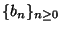is defined by the recurrence relation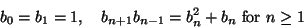and begins 1, 1, 2, 6, 21, 77, 286, 1066, ....

# Why is it interesting?

• It gives the numbers appearing in the number fence'' table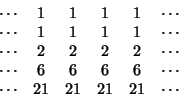where the top two rows are filled with ones and each number is the determinant of the matrix formed by the four numbers touching it. In other words, the matrix satisfies the recurrence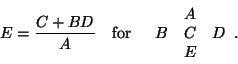It turns out that if we apply this recurrence relation to a table whose top two rows are filled with formal indeterminates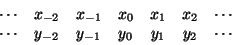then the entries in this table are (apparently) Laurent polynomials in the xj and yj in which every monomial has coefficient +1. Thus bn counts the number of monomials in this polynomial.

• It satisfies the relation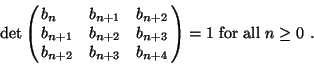(1)

This property is actually equivalent to the recurrence relation above; see David Speyer's writeup on the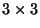determinant recurrence.

• We can write bn = cn cn+1, where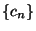is a new sequence defined by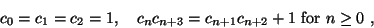which begins 1, 1, 1, 2, 3, 7, 11, 26, .... This sequence counts several things: for example, c2n is the number of domino tilings of a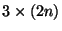rectangle. Again, see Speyer's writeup for more details.

• The sequence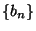also satisfies a linear recurrence

bn = 5bn-1 - 5bn-2 + bn-3

and hence has a rational generating function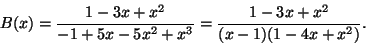# What is the combinatorial interpretation?

Construct an infinite ladder graph G shown below; then bn gives the number of paths in this graph from P0 to Pn moving only up and to the right (or moving to the left, in the drawing on the right).

               P_4     .
+-+ .
P_3  | |.                     P_0 P_1 P_2 P_3 P_4
+-+-+-+                     *   *   *   *   *
P_2  | |/| |                    / \ / \ / \ / \ / \
+-+-+-+-+                   *   *   *   *   *   *
P_1  | |/| |                      \ / \ / \ / \ / \ /
+-+-+-+-+                   ... *---*---*---*---* ...
P_0  | |/| |                        / \ / \ / \ / \ / \
+-+-+-+-+                       *   *   *   *   *   *
| |/| |                          \ / \ / \ / \ / \ /
+-+-+-+                           *   *   *   *   *
.| |
. +-+
.


# Why does it work?

I'll complete this section later, but for now, just an sketch of the proof: We prove thedeterminant relation (1) by applying the Gessel-Viennot Theorem (ref?) to the graph above with left endpoints (P0, P1, P2) and right endpoints (Pn+4, Pn+3, Pn+2). The key point is that there exists exactly one way of joining P0 to Pn+4, P1 to Pn+3 and P2 to Pn+2 by three vertex-disjoint paths in G. This determinant relation together with the initial cases b0 = b1 = 1, b2 = 2, b3 = 6 (which can be checked by hand) uniquely determine the sequence bn.

# What does it mean?

• The one-dimensional nature of the paths counted by bn makes it clear that the sequence, originally defined by a quadratic recurrence, will in fact satisfy a linear recurrence. Looking at the drawing of G on the left, if we define a 5-tuple pn as the number of paths from P0 to each of the five vertices on the row containing Pn, then there exists a fixed matrix M such that pn+1 = Mpn; the Cayley-Hamilton theorem then tells us that the pn satisfy a linear recurrence.

• As mentioned above, bn counts the monomials appearing in the Laurent polynomials given by the number fence'' recurrence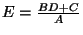; a combinatorial understanding of the sequenceis thus a natural first step in understanding the combinatorics of this recurrence relation.

Reid W Barton
2002-10-26